# 4.4 FEM solution for the two-dimensional Helmholtz equation

Finite element methods (FEM), initially developed for structural mechanics , are widely used for numerical simulations of electromagnetic fields , , , , . Some advantages of the finite element method are listed below.

• A sparse system matrix enables the simulation of problems with a large
number of unknowns.
• Suitable for inhomogeneous simulation domains.
• Adaptability to arbitrary geometries by triangular meshes for two-dimensional
simulations and tetrahedral meshes for three-dimensional problems.
• Suitable for inhomogeneous simulation domains.
• Existence of an unambiguous solution for elliptical partial differential equations.
This means convergence of the solution error towards zero with mesh refinement.

The finite element method provides an approximate solution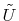for the elliptical partial differential equation (4.13). The residuum (approximation error) of this solution is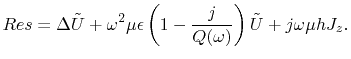(4.24)

For consistence to the voltage and current directions, defined by the impedance matrix in (4.17), a negative sign has been used for the current density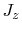, as opposed to the sign in (4.13). The residuum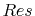, weighted with a function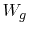, will vanish on average over the simulation domain. This is expressed in the variation integral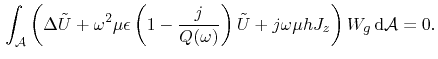(4.25)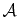denotes the whole simulation domain, that is, the parallel plane surface. Applying Green's theorem, (4.25) is transformed to the weak formulation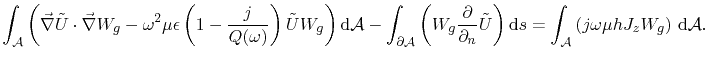(4.26)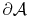is the boundary curve of the parallel plane surface,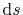is the line segment of this boundary curve andis the normal derivation ofat the boundary.is expressed by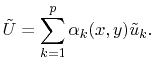(4.27)

Where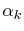denote the finite element base functions,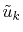are the values of the solution at the mesh points, and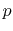denotes the number of mesh nodes. According to the method of Galerkin, the weighting function is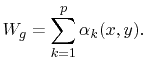(4.28)

Linear base functions on a triangular mesh are selected for the finite element discretization. Barycentric coordinates are utilized according to Figure 4.5.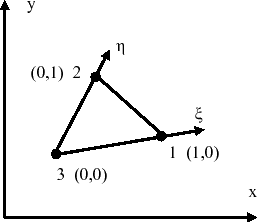Figure 4.5: Barycentric (triangular) coordinates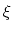and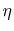. Coordinates (,) become (1,0), (0,1), and (0,0) in the nodes 1, 2, and 3, respectively.

With this coordinate system definition the base functions are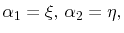and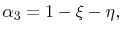(4.29)

with indexes according to the triangle node numbers in Figure 4.5. The relation of the barycentric to the cartesian coordinates of the triangular nodes is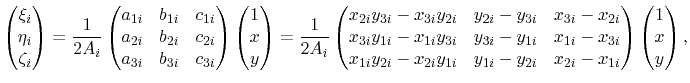(4.30)

with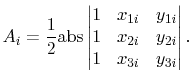(4.31)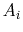is the surface area of the triangle with index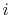. With equations (4.27) and (4.28), (4.26) becomes the linear equation system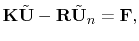(4.32)

for the voltages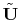on the mesh nodes.denotes the vector of the normal derivatives ofat the boundary. At open boundaries the PMC boundary condition requires vanishing. On closed metallic edges a Dirichlet boundary has to be introduced, by setting the boundary node voltage to zero and reducing the order of the linear system accordingly. Since the weighting function is set to zero in the boundary nodes with Dirichlet condition, the term withvanishes also at Dirichlet boundaries and (4.32) is reduced to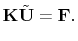(4.33)

The system matrix elements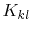are obtained from the solution of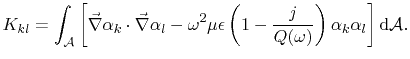(4.34)

The term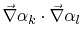in( 4.34) becomes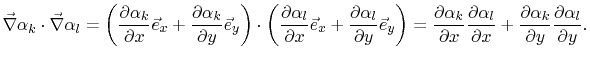(4.35)

With the base functions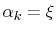and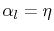(4.36)

and (4.30), the partial derivatives of (4.35) become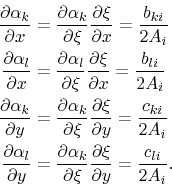(4.37)

Using these partial derivatives expressions (4.34) becomes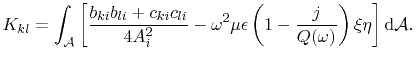(4.38)

Utilizing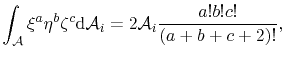(4.39)

from , the integral of (4.38) is solved analytically and the coefficients of the FEM matrixfinally become(4.40)

with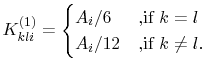(4.41)

At the node position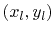the port excitation current is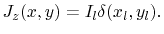(4.42)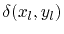is the Dirac pulse function and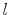is the node index running from one to the number of nodes. With (4.26), (4.28), (4.36), and (4.42), the coefficients of the excitation vector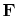in (4.33) become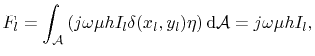(4.43)

because the weighting functionis unity at node positionaccording to the base function definition in (4.29). With this port current excitation definition the impedance matrix, which relates the voltages on the mesh nodes to the node excitation currents, is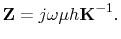(4.44)

Figure 4.6 depicts a comparison of the analytic results from (4.18) to the FEM results for the impedance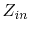of a port at positionon a rectangular cavity with dimensions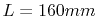,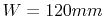and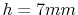. Although this FEM simulation was carried out using a very coarse mesh with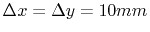, the results of this simulation match the analytical solution well. Thus, the proposed FEM method provides an efficient and accurate solution for arbitrarily shaped parallel plane cavities. In the case of a large number of excitation ports, the FEM method is even more efficient than the analytical solution. While the analytical method requires the calculation of the double sum term in (4.18) for each coefficient in the port impedance matrix (4.17), the FEM solution requires only one inversion of the sparse system matrix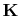. Linear base functions and a triangular mesh enable an efficient, analytical composition of the system matrixand the excitation vectorwith (4.40) and (4.43) respectively. Since a high accuracy is achieved with the linear base functions even on a coarse mesh, there is no need for higher order base functions. Table 4.2 contains a summary of the FEM equations.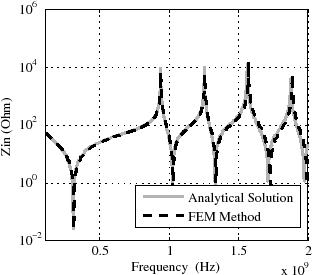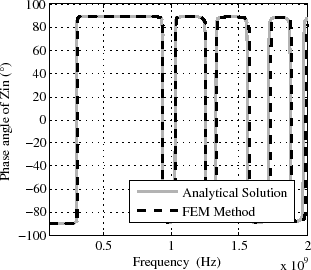(a) Magnitude comparison. (b) Phase angle comparison.
Figure 4.6: Comparison ofbetween the analytical solution (4.18) and the FEM solution. Cavity dimensions are (,,), the position of the port for theisand the mesh spacing is.

In the following the FEM equations are summarized.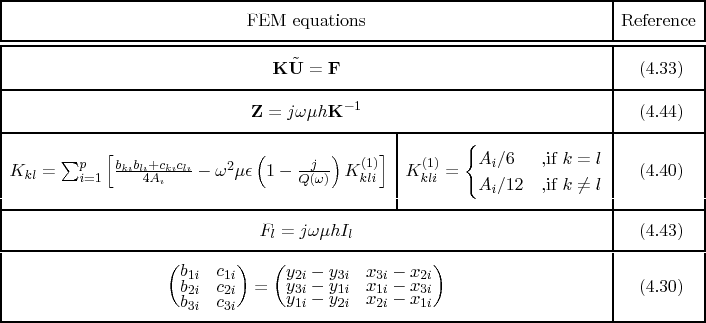C. Poschalko: The Simulation of Emission from Printed Circuit Boards under a Metallic Cover